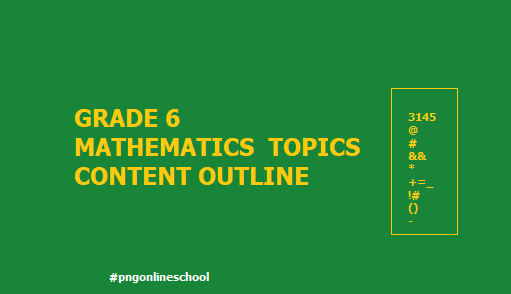# Grade 6 Mathematics Topics and Content outline

The following outlines  the yearly overview of the systematic plan of the grade 6 Mathematics content. It is helpful  for teachers, parents and students in the teaching and learning of mathematics at the Year 6 (Grade 6) level.  The strand is outlined and identifies each unit and topic into different strand groups. The units are in  sequential order from the first to the last unit.Grade 6 Mathematics Topics and Content outline

Those who are teaching the Grade 6 Mathematics can follow the content outline below.

Unit 1 Symmetry

•  Geometric Figures
• Shapes and Figures with Line Symmetry
• Shapes and Figures with Point Symmetry
• Polygons and Symmetry

Unit 2. Mathematical Letters and Expressions

• Data & Mathematical Relations
• Mathematical Letters and Expression
• Let’s Put Numbers into Mathematical Sentences

Unit 3. Multiplication of Fractions

• Number & Operation
• Operation of Fractions×Fractions
• Inverse of a Number

Unit 4. Division of Fractions

• Number & Operation
• Operation of Fractions÷Fractions
• What kind of Expression will it Become?

Unit 5. Multiples and Rates

• Data & Mathematical  Relations

Unit 6. Operation of Decimals and Fractions

• Number & Operation
• Operation of Decimal
• Operation of Fractions
• Operation of Fraction

Unit 7. Calculating the Area of Various Figures

• The Area of a Circle
• Approximate Area

Unit 8. Orders and Combinations

•  Ordering
• Combinations

Unit 9. Speed

• Speed
• Speed and Graphs

Unit 10. Volume

•  Volume of a Prism
•  Volume of a Cylinder

Unit 11. Ratio and its Application

• Ratio
•  Equivalent Ratio
•  Equivalent Ratio

Unit 12. Enlargement and Reduction of Figures

• Enlarging and Reducing Figures
• How to Draw Enlarged and Reduced Figures
• Uses of Reduced Figures

Unit 13. Proportion and Inverse Proportion

•  Proportion
•  Graphs of Proportion
•  Using the Properties of Proportion
•  Inverse Proportion

Unit 14. How to Explore Data
•  Mean
• How to Explore Distribution

Unit 15. Quantity and Unit

• How to Represent Quantity
•  Units of Length: km, m, cm, mm
• Units of Area: km², ha, a, m², cm²
• Units of Volume: m³, cm³, kL, dL, mL
• Units of Weight: t, kg, g, mg
• Metric System

Unit 16. Summary of Grade 3 to 6

• Numbers and Calculations
• Quantity and Measurement
• Shapes and Figures
• Data and Relations

Also check out